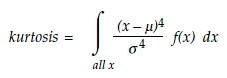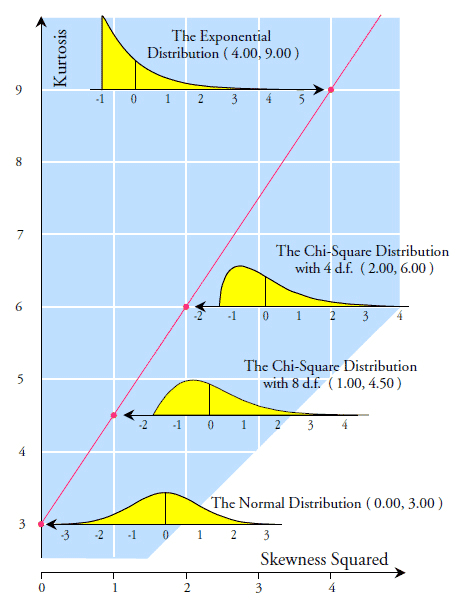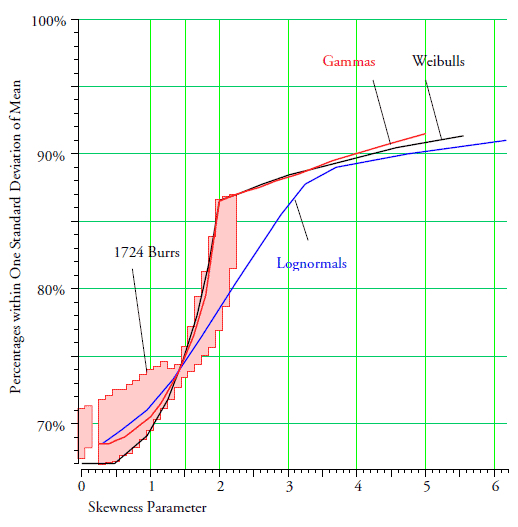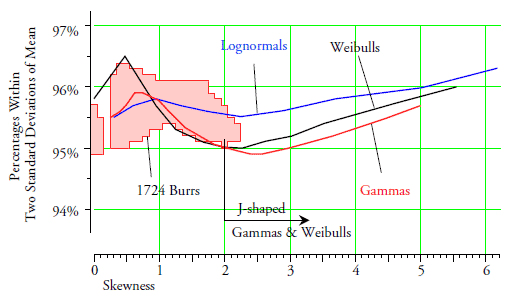#Quality Digest

Featured Product
This Week in Quality Digest Live
Statistics Features
Donald J. Wheeler
How you sample your process matters
Harish Jose
Using OC curves to generate reliability/confidence values
Donald J. Wheeler
The consequences of trying to fine-tune your charts
Donald J. Wheeler
The origin of the error function
Donald J. Wheeler
Using process behavior charts in a clinical setting
Statistics News
How to use Minitab statistical functions to improve business processes
New capability delivers deeper productivity insights to help manufacturers meet labor challenges
Day and a half workshop to learn, retain, and transfer GD&T knowledge across an organization
Elsmar Cove is a leading forum for quality and standards compliance
InfinityQS’ quality solutions have helped cold food and beverage manufacturers around the world optimize quality and safety
User friendly graphical user interface makes the R-based statistical engine easily accessible to anyone
Collect measurements, visual defect information, simple Go/No-Go situations from any online device
Good quality is adding an average of 11 percent to organizations’ revenue growth
Ability to subscribe with single-user minimum, floating license, and no long-term commitmentBio

Statistics

## Is There an Empirical Rule for Probability Models?

### What do distributions have in common?

Published: Monday, April 2, 2018 - 12:03

Last month we looked at what the empirical rule tells us about the data in a histogram. This month we will consider if there are any commonalities between different probability models that will allow us to make categorical statements without having to know the exact form of the probability model.

In order to work with multiple probability models we will need some systematic way to organize them relative to each other. We will need this organization in order to have a context for any generalizations we may make about probability models. The organizational device we shall use is the traditional one created by Karl Pearson more than 100 years ago—the shape characterization plane.

### The shape characterization plane

Instead of trying to organize drawings of many different probability density functions, Karl Pearson decided to use a more mathematical approach. He would organize collections of probability models using the “shape parameters” of skewness and kurtosis. By plotting these two parameter values as points in a plane he could show similarities and differences between distributions.

The skewness parameter for a probability model with density function f(x) is traditionally defined as:where µ and σ are the mean and standard deviation parameters for the probability model. The kurtosis parameter is defined as:Both skewness and kurtosis are weighted functions of a probability model f(x). The weights used are the standardized values for x raised to either the third or fourth power. By using standardized values as weights we end up with both the skewness and the kurtosis being independent of the mean and standard deviation of the probability model.

The shape characterization plane traditionally uses the squared skewness to define the x-coordinate and the kurtosis to define the y-coordinate for the point representing a particular distribution.

For example, all normal distributions have a skewness of zero and a kurtosis of three regardless of their mean or variance. So the shape characterization plane uses a single point at (0, 3) to represent the collection of all normal distributions.

Exponential distributions, regardless of their mean value, all have a skewness of two and a kurtosis of nine. Thus they are represented on the shape characterization plane by a point at (4, 9).

The chi-square distribution with 4 degrees of freedom has a skewness of 1.4142 and a kurtosis of 6.000. Thus, it is represented by a point at (2, 6) on the shape characterization plane.

The chi-square distribution with 8 degrees of freedom has a skewness of one and a kurtosis of 4.50 and is represented by a point at (1.00, 4.50) on the shape characterization plane. The standardized forms of these density functions are shown in figure 1 along with their corresponding points in the shape characterization plane.Figure 1: Four distributions on the shape characterization plane

The line in figure 1 represents the family of all gamma distributions. Since all chi-square distribution are members of the family of gamma distributions, every chi-square distribution will have a point that falls on the line shown. By collecting together the points that represent a given family of distributions the shape characterization plane makes these families visible in a compact and efficient manner. It also allows us to see how different families of distributions are related. For example, figure 2 shows curves which represent the family of positively skewed Weibull distributions and the family of lognormal distributions.Figure 2: Mound-shaped distributions

The 1,724 pink points in figure 2 represent the family of Burr distributions. These distributions generally fall above the curve representing the Weibull distributions. They are all mound-shaped. To illustrate the wide range of shapes in the Burr family 18 of these distributions have their density functions shown. The Burr distributions extend up above the region shown in figure 2, and they essentially fill up most of the region of mound-shaped distributions. Because of this wide and almost complete coverage, we will use the Burr distributions along with the gammas, Weibulls, and lognormals in our search for empirical rules for probability models.

### Areas within one standard deviation of the mean

With a histogram we expect to find roughly 60 percent to 75 percent of the data within one standard deviation of the average. So in our search for empirical rules for probability models we begin with the areas within one standard deviation of the mean. These areas are shown graphically in figure 3.Figure 3: Areas within one standard deviation of the mean

The areas within one standard deviation of the mean for the 1,724 Burr distributions are shown by the shaded region. The areas for the Weibull, gamma, and lognormal families are shown by the three curves. Over these four families of distributions we find areas ranging from 67 percent to 91 percent.

There is however, a curious pinch point when the skewness is 1.5. Distributions with less skewness will have less than 75 percent within one standard deviation of the mean. Distributions with greater skewness will have more than 74 percent within one standard deviation of the mean. It is as if we could draw a vertical line at a squared skewness of 2.25 in figure 2 and characterize the areas within one standard deviation of the mean according to which side of the line the distribution fell.

The knee points seen on the Weibull and gamma curves at a skewness of 2.0 mark the cross-over from mound-shaped gamma and Weibull models on the left to J-shaped gamma and Weibull models on the right. The gamma curve continues on up to a skewness of 5 and a kurtosis of 40; the Weibull curve continues on up to a skewness of 5.5 and a kurtosis of 60; while the lognormal curve goes up to a skewness of 6.2 and a kurtosis of 114. Thus the region covered by figure 3 far exceeds the region shown in figure 2.

While there is a definite structure to the areas within one standard deviation of the mean, the fact that these areas range from 67 percent to more than 91 percent makes any attempt to come up with a categorical statement about these areas either overly complicated or unhelpfully vague.

### Areas within two standard deviations of the mean

With a histogram we expect to find usually 90 percent to 98 percent of the data within two standard deviations of the average. So in our search for empirical rules for probability models we next look at the areas within two standard deviations of the mean. These areas are shown graphically in figure 4.Figure 4: Areas within two standard deviations of the mean

Once again the shaded region shows the areas for the 1,724 Burr distributions. These areas range from a minimum of 0.949 to a maximum of 0.963, which is a remarkably small range of values. More than 1,700 probability models, with a huge range of shapes, all have basically 95 percent to 96 percent of their area within two standard deviations of the mean!

The curve for the gammas stays above 0.949. The curve for the Weibulls stays above 0.950. And the curve for the lognormals stays above 0.955. Once again starting with distributions near the normal, and moving out to distributions with kurtosis values of 40, 60, and over 100, we find that all of these distributions have between 94.9 percent and 96.5 percent of their areas within two standard deviations of the mean!

So here we find a very strong characterization that applies to virtually all probability models: Regardless of the family of probability models, mound-shaped and J-shaped distributions will effectively have 95 percent to 96 percent of their area within two standard deviations of the mean!

### Areas within three standard deviations of the mean

With a histogram we expect to find approximately 99 percent of the data within three standard deviations of the average. In our search for empirical rules for probability models we consider the areas within three standard deviations of the mean. These areas are shown graphically in figure 5.Figure 5: Areas within three standard deviations of the mean

As the skewness increases we see a general decline in the areas within three standard deviations of the mean, but there is a limit to this decline. For mound-shaped distributions there will always be at least 98 percent within three standard deviations of the mean. We see this with the 1,700 Burrs, all the lognormal distributions, the mound-shaped gammas, and the mound-shaped Weibulls.

Moreover, all J-shaped Weibulls will have between 97.8 percent and 98.2 percent within three standard deviations of the mean. All J-shaped gammas will have between 97.6 percent and 98.2 percent.

This is yet another strong result. Without specific knowledge of which probability model is appropriate, we can categorically say that we will find approximately 98 percent or more within three standard deviations of the mean.

### Summary

So there are certain percentages to be found within two and three standard deviations of the mean across the class of mound-shaped distributions and the commonly used J-shaped distributions. While the wide range of areas found within one standard deviation of the mean precludes any strong generalizations, we know that the bulk of the area will be found here, and surprisingly, the core of a distribution becomes more massive as its skewness increases.Figure 6: What we can say about probability models

In contrast to the above, regardless of which of our probability models we choose, we will find 95 percent to 96 percent of the area within two standard deviations of the mean!

For mound-shaped distributions at least 98 percent of the area will fall within three standard deviations of the mean. For commonly encountered J-shaped distributions at least 97.6 percent of the area will fall within three standard deviations of the mean. Thus, regardless of the probability model, you will never find more than about one or two percent outside three standard deviations from the mean.

So there are a couple of useful empirical rules for probability models. These rules simplify the job of making sense of our data. Understanding these simple rules and how they characterize probability models can help you to avoid logical fallacies that frequently result in misunderstandings when interpreting data.

If you would like to work with the family of Burr distributions used here, I can send you a pdf file that summarizes the Burr distributions (along with the formulas needed) and an Excel file with the parameter values needed to reproduce the 1,724 Burr distributions of figure 2.

### Discuss